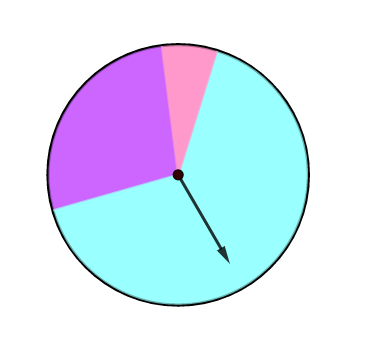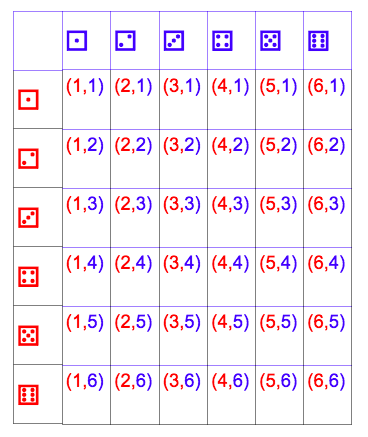Experimental & Theoretical Probability
starstarstarstarstarstarstarstarstarstar
by Hannah Ryan
| 22 Questions
Note from the author:
Statistics1
1 pt

Pink
Purple
Blue

# Spin the wheel 10 times and record how many times the spinner lands on each color.

The outlined content above was added from outside of Formative.
2
1 pt

3
1 pt

4
1 pt

# Drag the movable points below to display how likely it was for the spinner to land on each color.

The outlined content above was added from outside of Formative.5
1 pt

(Select all)
A
B
C
D

# The Probability of an Event can be written as a ratio, decimal, or percent.6
1 pt

# So the theoretical probability of flipping Heads is 1:2, ___ /___, or ______%.

[Separate your answers with commas & Simplify Fractions]

# Theoretical Probability is what is expected to happen based on the possible outcomes, assuming equally likely events.

"What you expect to happen, but isn't always the case."

# Flip the coin below 10 times and record the number of times you land on Heads.

The outlined content above was added from outside of Formative.
7
1 pt

# Experimental Probability is the result of an experiment or simulation after a large number of times.

"The probability determined from the results of an experiment."
8
1 pt

# What is the experimental probability fraction?

# Heads / Total # of Flips

[Simplify Fraction]
9
1 pt

# What is the experimental probability ratio?

# Heads : # Total Flips

[Simplify your answer]

# Roll the die ten times and record the numbers in the table.

1st: Click "Reset" and count the number you see for Trial #1
2nd: press "Roll" to view Trials 2 - 10.
The outlined content above was added from outside of Formative.10
11
12
13
14
15
10
1 pt
How many times did the #1 occur?
What is the Exp. Prob. Fraction?
11
1 pt
How many times did the #2 occur?
What is the Exp. Prob. Fraction?
12
1 pt
How many times did the #3 occur?
What is the Exp. Prob. Fraction?
13
1 pt
How many times did the #4 occur?
What is the Exp. Prob. Fraction?
14
1 pt
How many times did the #5 occur?
What is the Exp. Prob. Fraction?
15
1 pt
How many times did the #6 occur?
What is the Exp. Prob. Fraction?
16
1 pt

# What do you think will happen to the Experimental Probability if you were to increase the number of times you rolled the die?

The experimental probability would move farther from the theoretical probability.
The experimental probability would reach closer to the theoretical probability.
17
1 pt

# Predict how many times you rolled a 3 or a 6.

Exactly 200 times.
Around 200 times.
18
1 pt

# What is the Theoretical Probability that you roll a number less than 3.

[Select all that apply]
2/6
3/6
1/3
1/2
≈ 33%
≈ 50%

# Use the table below to find the probability of rolling 2 dice that add to 7.19
1 pt

20
1 pt

# P(rolling a sum of 7) = ?/36

[Write your answer in simplified fraction form]

# 24 have brown hair.21
1 pt

# What is the probability a person has blonde or black hair?

[Write your answer in fraction form]
22
1 pt

# Find the probability that a person has neither brown nor black hair.

[Write your answer in fraction form]
Add to my formatives list